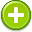#inequality 中文解釋 wordnet sense Collocation Usage
Noun
/ˌiniˈkwälitē/,Font size:inequalities, plural;
1. Difference in size, degree, circumstances, etc.; lack of equality
• - social inequality
• - the widening inequalities in income
2. Lack of smoothness or regularity in a surface
• - the inequality of the ground hindered their footing
3. The relation between two expressions that are not equal, employing a sign such as ≠ “not equal to,” > “greater than,” or < “less than.”

4. A symbolic expression of the fact that two quantities are not equal

1. lack of equality; "the growing inequality between rich and poor"
2. In mathematics, an inequality is a statement about the relative size or order of two objects, or about whether they are the same or not (See also: equality). *The notation a < b means that a is less than b. *The notation a > b means that a is greater than b. ...
3. An unfair, not equal, state; A statement that of two quantities one is specifically less than (or greater than) another. Symbol: < or ≤ or > or ≥, as appropriate
4. (Inequalities) A sentence that contains > (is greater than), < (is less than), = ( is less than or equal to), or (is not equal to). Such sentences are of the following forms:
5. (8. Inequalities) This program teaches students the properties and solution of inequalities, linking positive and negative numbers to the direction of the inequality. The program presents three applications of inequalities: modeling problems of the U.S. ...
6. Inequalities can lead to mathematical fallacies when operations that fail to preserve the inequality [citation needed] are not recognized. Let us first suppose that
7. An inequality is like an equation that uses symbols for "less than"(<) and "greater than"(>) where an equation uses a symbol for "is equal to" (=). ...
8. a mathematical sentence that includes one of the symbols >, <, or
9. A mathematical expression which shows that two quantities are not equal.
10. State or condition of being unequal. Amartya Sen argues that virtually all political philosophies want equality of something something that has an important place in the particular theory (Sen 1992: ix). Libertarians want equal rights; others demand equal welfare or incomes. ...
11. Being unequal. Not being allowed the same rights or opportunities as everyone else in the community.
12. Differences in per capita income or household income across populations within a country or across countries.
13. Inequality implies that the distribution of social resources is concentrated more upon some than upon others and may be either justified (if this distribution is based upon efforts, choice and merit) or unjustified (if based upon relations of oppression, discrimination and class).
14. refers to the condition of being unequal or social or economic disparity.
15. Differences between people or groups in terms of power, status, and/or access to resources.
16. Two expressions, separated by an inequality sign (< or >). For example, y > x + 3 is an inequality. The > sign means "greater than."
17. Rawls          . [JR/JF/55]      “any modern society, even a well-ordered one, must rely on some inequalities to be well designed and effectively organized…. ...
18. a statement in which the relationships are not equal. The opposite of an equation.
19. not equal in size, amount or value
20. A mathematical expression containing a greater than or equal to relation (≥) or a less than or equal to relation (£) between the left-hand side and the right-hand side of the expression.
21. NOT EQUAL (1x) SENTENCE.